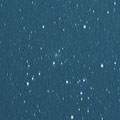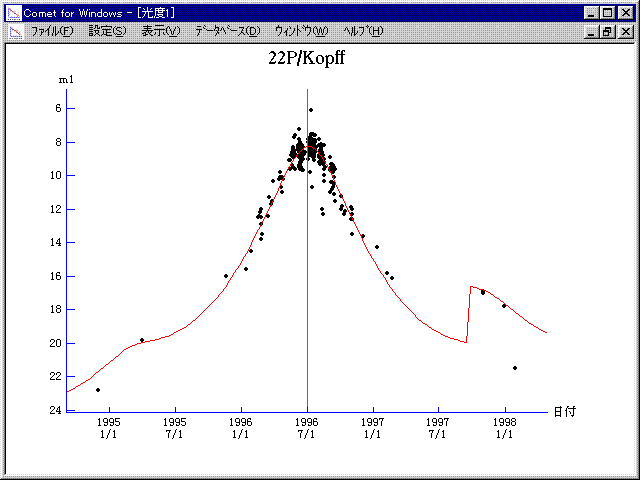# \$B%3%W%UWB@1(B

22P/Kopff (1996)###\$B###\$B50F;MWAG(B

```   The following improved orbital elements by Kenji Muraoka,
are from 539 observations 1982 to 1997, including 9 Planets,
Moon and 5 minor planets perturbations and non-gravitational
effect of style II.  The mean residual is +/- 0.93 arc seconds.

Epoch  =  1996 July 16.0  TT       JDT = 2450280.5
T  =  1996 July  2.19151       +/- 0.00034 (m.e.) TT
Peri. =  162.83733                +/- 0.00021
Node  =  120.90697                +/- 0.00009   (2000.0)
Incl. =    4.72102                +/- 0.00001
q  =    1.5795751              +/- 0.0000006 AU
e  =    0.5440721              +/- 0.0000003
a  =    3.4645283              +/- 0.0000007 AU
n  =    0.15284031             +/- 0.00000005
P  =    6.449                  +/- 0.0000021  years
A1  =   -0.161                  +/- 0.016
A2  =   -0.09090                +/- 0.00088
```

###\$B@1?^(B1995\$BG/(B 5\$B7n(B 3\$BF|!A(B1996\$BG/(B 9\$B7n(B24\$BF|(B1996\$BG/(B 4\$B7n(B27\$BF|!A(B1997\$BG/(B 8\$B7n(B20\$BF|(B

###\$B8wEYJQ2=(B

```        m1 = 5.3 + 5 log\$B&\$(B + 21.0 log r  [   ,450]  (              \$B!A(B1997\$BG/(B 9\$B7n(B25\$BF|(B)
m1 = 2.5 + 5 log\$B&\$(B + 20.0 log r  [450,   ]  (1997\$BG/(B 9\$B7n(B25\$BF|!A(B              )
```##### \$B50F;MWAG\$OB<2,7r<#;a\$N7W;;\$K\$h\$k\$b\$N\$G\$9!#(B \$B@1?^\$O(B StellaNavigator Ver.2.0 for Windows (\$B%"%9%H%m%"!<%D(B \$BJTCx(B / \$B%"%9%-!<=PHG6I4)(B) \$B\$G:n@.\$7\$?\$b\$N\$G\$9!#(B \$B8wEY%0%i%U\$O(BComet for Windows\$B\$G:n@.\$7\$?\$b\$N\$G\$9!#(B